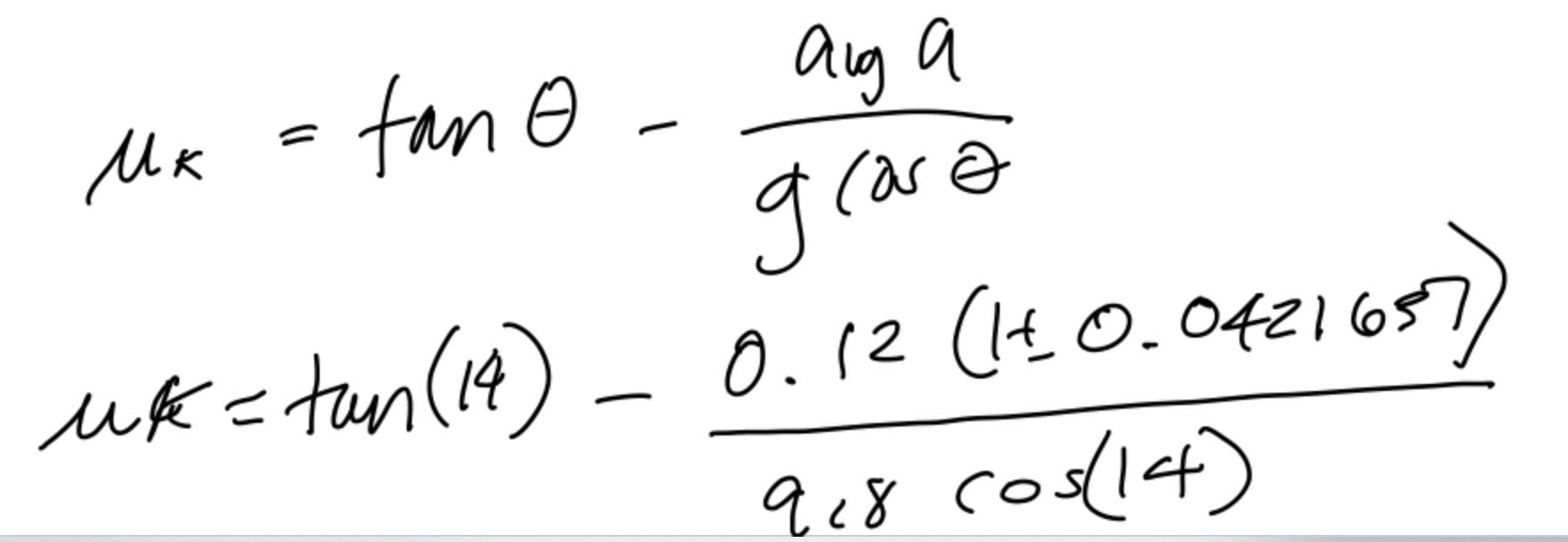# Determine the coefficent of kinetic friction, μk, with error due to uncertainty in average acceleration? Using the below equation and values.  μk= tan θ - (average acceleration (1+-0.0421637)/9.8 cos θ) Attached below is equation.

Question

Determine the coefficent of kinetic friction, μk, with error due to uncertainty in average acceleration? Using the below equation and values.

μk= tan θ - (average acceleration (1+-0.0421637)/9.8 cos θ)

Attached below is equation.fullscreen

## Expert Answer

### Want to see this answer and more?

Experts are waiting 24/7 to provide step-by-step solutions in as fast as 30 minutes!*

*Response times may vary by subject and question complexity. Median response time is 34 minutes for paid subscribers and may be longer for promotional offers.
Tagged in
Science
Physics© 2021 bartleby. All Rights Reserved.